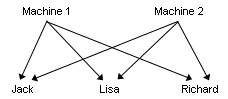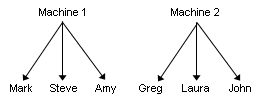# Types of factors in a gage R&R study

## What are crossed and nested factors?

Two factors are crossed when each level of one factor occurs in combination with each level of the other factor.For example, if you use crossed factors in your study, the same three operators would inspect surface finish from both machines.
Two factors are nested when the levels of one factor are similar but not identical, and each occurs in combination with different levels of another factor.For example, if Machine 1 is at one location and Machine 2 is at a different location, each machine will have different operators.

## What are fixed and random factors?

Categorical factors can be either fixed or random. Usually, if the investigator controls the levels of a factor, then the factor is fixed. Conversely, if the investigator randomly sampled the levels of a factor from a population, then the factor is random.

Suppose you have an operator factor that has three levels. If you intentionally select three operators and want your results to apply to only these operators, then the factor is fixed. However, if you randomly sample three operators from a larger number of operators, and you want your results to apply to all operators, then the factor is random.

## Should I use a crossed, nested, or expanded gage R&R study?

Use the gage R&R study that is designed for the type and number of factors that you have.
Crossed gage R&R study
In a crossed study, each operator measures each part. This study is called crossed because the same parts are measured by each operator multiple times. To perform a crossed gage R&R study in Minitab, choose Stat > Quality Tools > Gage Study > Gage R&R Study (Crossed).
Often, you will use a crossed gage R&R study to determine how much of your process variation is due to measurement system variation.
Nested gage R&R study
In a nested study, each part is unique to the operator; no two operators measure the same part. This study is called nested because one or more factors is nested under another factor and, thus, not crossed with the other factors. To perform a nested gage R&R study in Minitab, choose Stat > Quality Tools > Gage Study > Gage R&R Study (Nested).
Expanded gage R&R study
Use an expanded study when one or more of the following conditions exists:
• You have more than two factors, for example, operator, gage, and part.
• You want to declare some factors as fixed factors.
• You have both crossed and nested factors.
• You have an unbalanced design.
This study is called expanded because you can use it in several types of situations. To perform an expanded gage R&R study in Minitab, choose Stat > Quality Tools > Gage Study > Gage R&R Study (Expanded).
By using this site you agree to the use of cookies for analytics and personalized content.  Read our policy